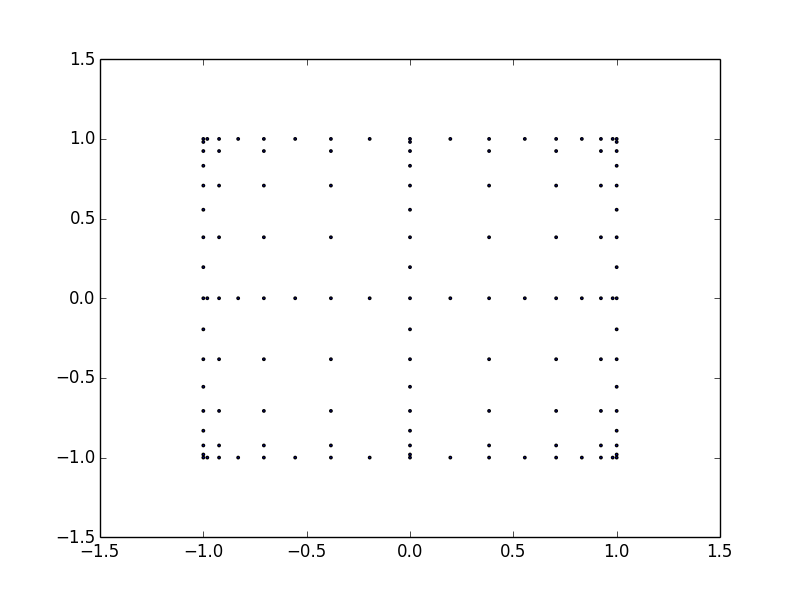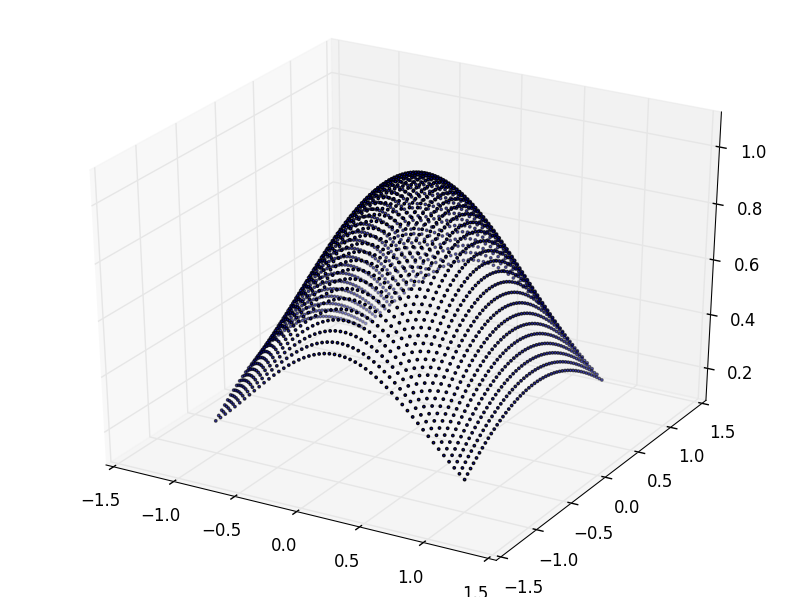View on GitHub

# py_tsg

## A Python wrapper around the Tasmanian sparse grid library

Project page: http://rncarpio.github.com/py_tsg

### What is `py_tsg`?

`py_tsg` is a Python wrapper around the Tasmanian Sparse Grid Library (TSG) for high-dimensional interpolation and integration using sparse grids (e.g. Smolyak grids). TSG is written in C++ and supports a variety of polynomial basis functions, anisotropic weights, and adaptive local refinement. For more information on TSG, visit http://tasmanian.ornl.gov/ or look at the manual.

This version is based on TSG v1.0, released August 2013. The code has been slightly modified to compile under Windows. The Python wrapper requires Boost.Python and PyUblas to be installed.

### Installation

Assuming you have Boost.Python and PyUblas already installed, download the files and type `make all`. The makefile should handle both Linux and Windows (tested with MSVC 2010). A shared library `libtasmaniansparsegrid.so` or its Windows equivalent should be produced; set your paths to detect this. Another shared library `_py_tsg.so` or `_py_tsg.pyd` should be produced; from Python, you can type `import _py_tsg`.

### Interface

The interface is a straightforward translation of the C++ API. See the TSG manual and the file `tsg_python.cpp` for details.

### Examples

The following examples are copied from the `example.cpp` file in the TSG distribution. Example 1:

```# EXAMPLE 1:
# make a classical Smolyak grid using Clenshaw-Curtis quadrature
# integrate the function f(x,y) = exp( -x^2 ) * cos( y )
# the exact answer is: 2.513723354063905e+00

import scipy, scipy.integrate, itertools
import pyublas
import _py_tsg as tsg

grid = tsg.TSG()
def fn1(x):     return scipy.exp(-x*x)*scipy.cos(x)
dimension = 2
outputs = 0
level = 7
grid.make_global_grid( dimension, outputs, level, tsg.TypeDepth.type_level, tsg.TypeOneDRule.rule_clenshawcurtis, scipy.array([], dtype=int), 0, 0 )
points = grid.get_points()
weights = grid.get_weights()
sum = scipy.sum( [w*fn1(x) for (x,w) in zip(points, weights)] )
print("\nExample 1")
print("grid has: %d points" % grid.get_num_points())
print("integral is: %.17f" % sum)
print("error: %.17f" % scipy.fabs( sum - 2.513723354063905e+00 ))
```

produces:

``````Example 1
grid has: 321 points
integral is: 2.51372335405531810
error: 0.00000000000858691
``````

Example 2:

```# make a Clenshaw-Curtis rule that interpolates exactly polynomials of order up to 10
# integrate the function f(x,y) = exp( -x^2 ) * cos( y )
# exact values is: 6.990131267703512e-01

import scipy, scipy.integrate, itertools
from mpl_toolkits.mplot3d import Axes3D
import matplotlib.pyplot as plt

import pyublas
import _py_tsg as tsg

def apply_fn_to_grid(gridList, fn):
xy_list = list(itertools.product(*gridList))
xlists = []
[xlists.append( [xy[i] for xy in xy_list] ) for i in range(len(gridList))]
f_list = [fn(xy) for xy in xy_list]
return (xlists, f_list)

def plot_gridpoints_2(grid):
assert(grid.get_num_dimensions() == 2)
points = grid.get_points()
fig = plt.figure()
plt.scatter(points[:,0], points[:,1], s=3)
print("%d gridpoints" % len(points))

def scatter_grid_2(grid, n_gridpoints=20):
assert(grid.get_num_dimensions() == 2)
(low, high) = grid.get_transform_AB()
grid1 = scipy.linspace(low, high, n_gridpoints)
grid2 = scipy.linspace(low, high, n_gridpoints)
(xlists, f_list) = apply_fn_to_grid([grid1, grid2], lambda x: grid.evaluate(scipy.array(x)))
fig = plt.figure()
ax = Axes3D(fig)
ax.scatter3D(xlists, xlists, f_list, s=3)
return ax

grid = tsg.TSG()
def fn1(x):     return scipy.exp(-x*x)*scipy.cos(x)
dimension = 2
outputs = 1
precision = 10
grid.make_global_grid( dimension, outputs, precision, tsg.TypeDepth.type_basis, tsg.TypeOneDRule.rule_clenshawcurtis, scipy.array([], dtype=int), 0, 0 )
points = grid.get_needed_points()
values = fn1(points.T)
produces`py_tsg` is free software: you can redistribute it and/or modify it under the terms of the GNU General Public License as published by the Free Software Foundation, either version 3 of the License, or (at your option) any later version.